# Capacitance – Capacitance of two parallel plates

0
4224

### Electrical Capacitance

You must have heard of the word capacity, for example the capacity of the stadium, or the capacity of the lungs. The word stadium capacity means: How many stadiums can visitors receive. so, by analogy, the word electrical capacity of conductors could mean what quantity charge conductor can receive.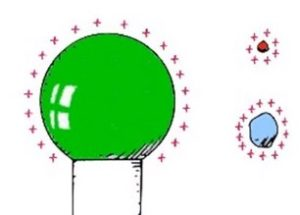Capacitance is the ability to store electrical charge. The measure of the ability of a conductor to store sharges is known as capacitance or capacity.  If you change the amount of electricity on the conductor, the potential of the conductor is also changing. The relationship between q and V on the conductor is constant.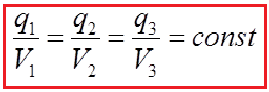Capacitance of a conductor is defined as the charge required to raise its potential through one unit.

The conductor capacity is equal to the amount of charge and i achieved electric potential.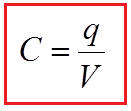It is sometimes easier to remember this relationship by using pictures.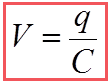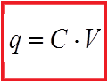Here the three quantities of Q, C and V.Where: Q (Charge, in Coulombs) = C (Capacitance, in Farads) x V (Voltage, in Volts).

SI Unit of capacitance is „farad“ (F). Capacitance is said to be 1 frad  when 1 coulomb of charge raises teh potential  of conductor by 1 volt. Since 1 coulomb is the big of charge, the capacitance be usually in the range of mili fard, micro farad, nano farad or pico farad. Even the Earth has no capacity of 1 farad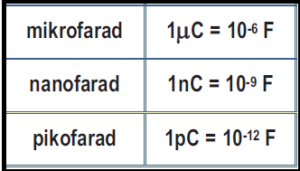Example 1: If an isolated metal ball is connected to the electrometer, the amount of charge is charged. The electrometer will show a greater deviation when the amount of charge is higher.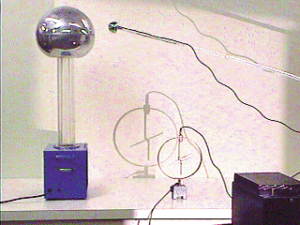## Capacitors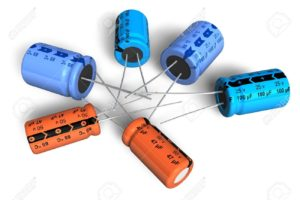Capacitors are the most widely used electronic components. We find capacitors in televisions, computers, and all electronic circuits. A capacitor is an electronic device that stores electric charge or electricity when voltage is applied and releases stored electric charge whenever required.

### First Capacitor-Leyden jar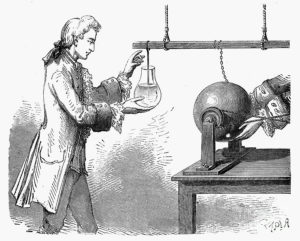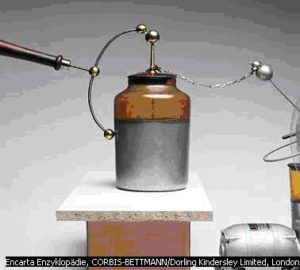Leyden jar – The Leyden jar is an early capacitor that was invented independently by a German cleric Ewald Georg von Kleist and by Pieter van Musschenbroek of Leyden, Holland around 1745. It is important because it was used in many of the early experiments in electricity, including experiments performed by Benjamin Franklin. It consists of a glass jar coated with metal foil about halfway up on both inside and outside. A brass ball and rod passing down through an insulating top is electrically connected to the inner metal foil by a metal chain as shown in Fig. The jars were also filled half full of water. To charge a Leyden jar, the brass knob is connected to one terminal of a static charge generator and the outer coating is grounded, as by holding the jar in your hand.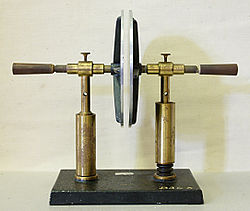In one place in the Bible, the details describe the machine with great precision in which electricity generated by friction of air on silk curtains and was packed in a box constructed as a capacitor.

## Applications of Capacitors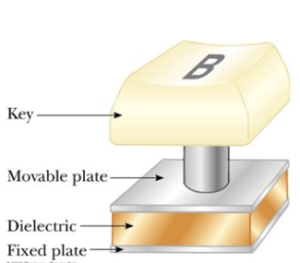### Applications of Capacitors

Computers Computers use capacitors in many ways Some keyboards use capacitors at the bases of the keys When the key is pressed, the capacitor spacing decreases and the capacitance increases The key is recognized by the change in capacitance

###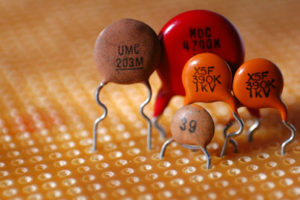The Capacitor, sometimes referred to as a Condenser, is a simple passive device that is used to “store electricity”.

The capacitor is a component which has the ability or “capacity” to store energy in the form of an electrical charge producing a potential difference (Static Voltage) across its plates, much like a small rechargeable battery.

The property of a capacitor to store charge on its plates in the form of an electrostatic field is called the Capacitance of the capacitor. Not only that, but capacitance is also the property of a capacitor which resists the change of voltage across it.

The capacitors ability to store this electrical charge ( Q ) between its plates is proportional to the applied voltage, U.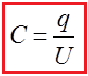Note that capacitance C is ALWAYS positive and never negative.

### What is the principle of capacitor?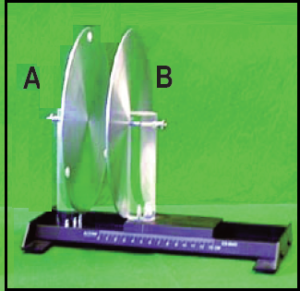Operation of a capacitor. Let us connect a electroscope to a metal plate, as shown picture, and place a charge on the plate. As we increase the charge, the leaves of the electroscope will diverge more and more widely, and the potential of the plate will increase in proportion to the charge (the potential of any charged plate must be proportional to the amount of charge on the plate). At some point the plate will reach the point where the electrons will leak to the air as rapidly as they are supplied and the plate will be at capacity. Let us now bring a second plate, plate B, which is grounded (connected to the ground), close to it as shown in picture  (b). As we bring plate B close to it, the leaves of the electroscope start to fall together. The closer we bring plate B, the further the leaves fall. As the leaves fall, the potential on plate A falls also, and the plate’s capacity for holding charge increases. We find we can now add a lot more charge to the plate before it reaches capacity.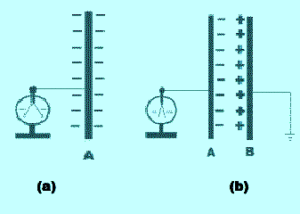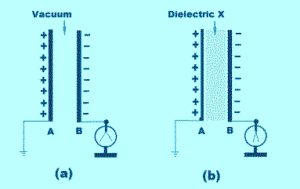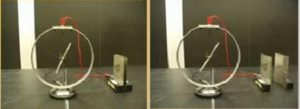What happened here? As we brought plate B close to plate A, the amount of charge on plate A remained the same. Why did the leaves on the electroscope fall? Answer: When plate B was brought opposite to plate A, it became charged by induction. Its electrons were repelled to the far side of the plate and away to the earth through the ground wire. The unbalanced positive charges remaining on plate B acted to “bind” the electrons on plate A, neutralize them, and reduce its potential. With the presence of the unbalanced positive charges on plate B, it takes less work to place the same negative charge on plate A which, reduces its potential.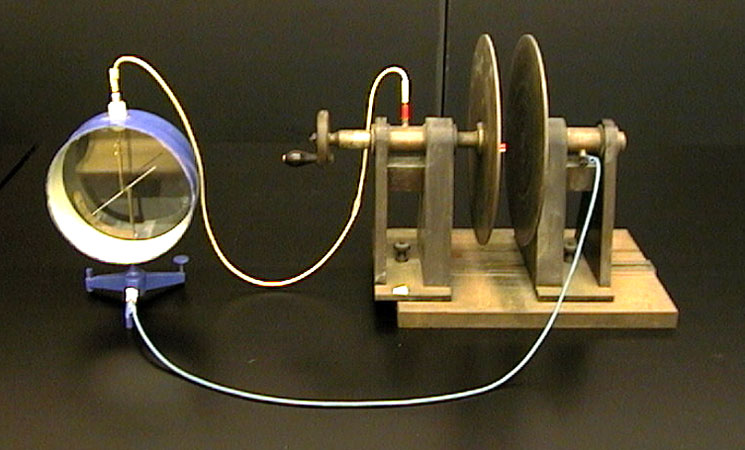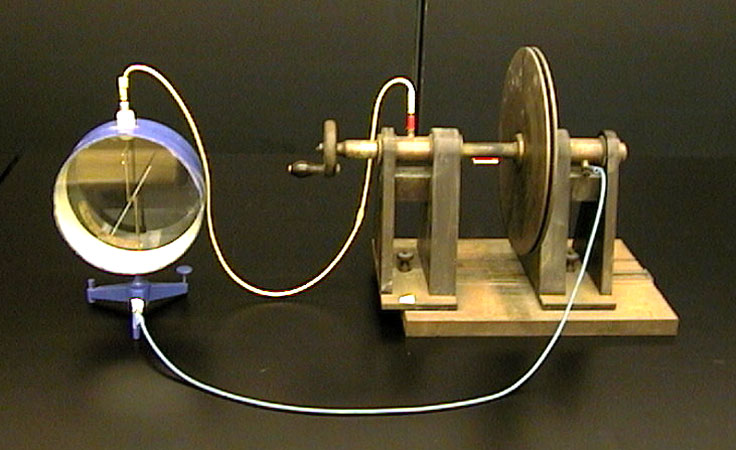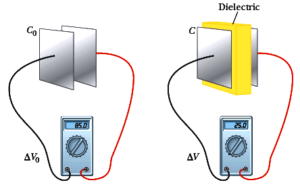Storage of electricity in capacitors

Storing Energy in a Capacitor Capacitors can be used to store electrical energy. The amount of energy stored is equal to the work done to charge it. During the charging process, the battery does work to transfer charges from one plate and deposit them onto the other. Figure : Work is done by an external agent in bringing +q (or battery) from the negative plate and depositing the charge on the positive plate.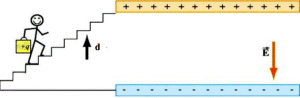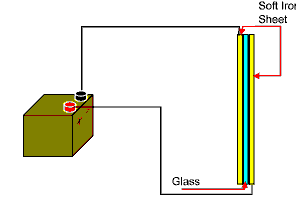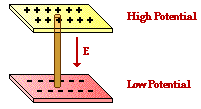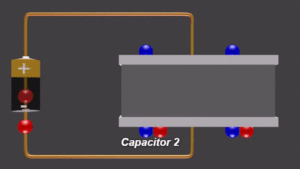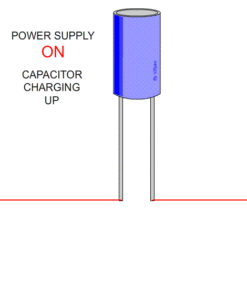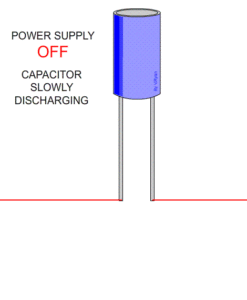Different types of electrical capacitors: multilayer ceramic, disc-ceramic, multilayer foil, tubular ceramic, polystyrene (axial and radial), electrolytic.### Construction of a capacitor

The basic construction of all capacitors is similar. The construction of capacitor is very simple. A capacitor is made of two electrically conductive plates placed close to each other, but they do not touch each other. These conductive plates are normally made of materials such as aluminum, brass, or copper. The construction of capacitor is very simple.

A capacitor is made of two electrically conductive plates placed close to each other,The empty space between these plates is filled with a non-conductive material or electric insulator or dielectric region. The non-conductive material or region between the two plates may be an air, vacuum, glass, liquid, or solid. This non-conductive material is called dielectric.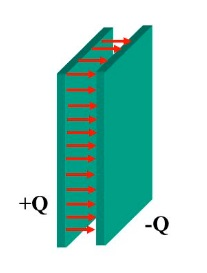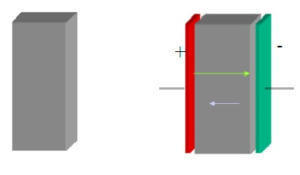When a capacitor is charged, its plates have charges of equal magnitudes but opposite sign: +q i –q. However, we refer to the charge of a capacitor as being, the apsolute value of these charges on the plates.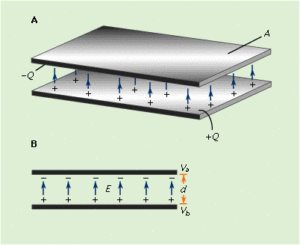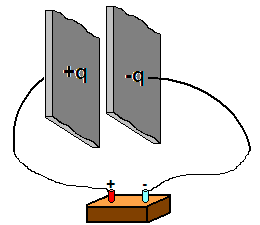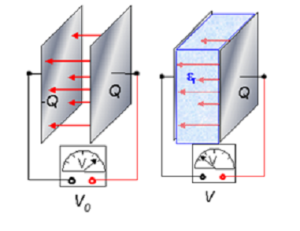In general a capacitor’s capacity for holding charge increases with the area of the plates and increases as the distance between the plates decreases.

### Capacitance of two parallel plates

A simple demonstration capacitor made of two parallel metal plates, using an air gap as the dielectric.

A capacitor is just two plates spaced very close together, and it’s basic function is to hold a whole bunch of electrons. The greater the value of capacitance, the more electrons it can hold. If the size of the plates is increased, the capacitance goes up because there’s physically more space for electrons to hang out. And if the plates are moved farther apart, the capacitance goes down, because the electric field strength between them goes down as the distance goes up.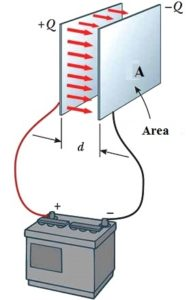The capacitance of a capacitor is a number representing a measure of the capacitor’s storage capability. It is a constant for a given capacitor. For example, the capacitance of a parallel plate capacitor (the most common type of capacitor and the type we considered in the above experiment) is given by the formula:where

A = area of the plates (m2)

d = distance between the plates (m)

ε = dielectric constant for the dielectric between the plates

ε0 = 8.85×10-12 C2/N-m2

###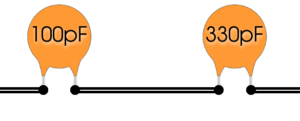Series and Parallel Capacitors

Capacitors are one of the standard components in electronic circuits. Moreover, complicated combinations of capacitors often occur in practical circuits. It is, therefore, useful to have a set of rules for finding the equivalent capacitance of some general arrangement of capacitors. It turns out that we can always find the equivalent capacitance by repeated application of two simple rules. These rules related to capacitors connected in series and in parallel.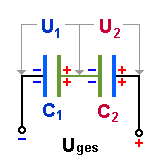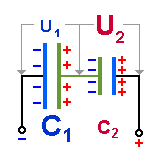When capacitors are connected in series, the total capacitance is less than any one of the series capacitors’ individual capacitances. If two or more capacitors are connected in series, the overall effect is that of a single (equivalent) capacitor .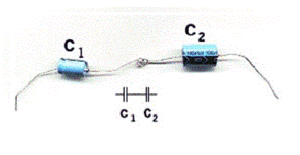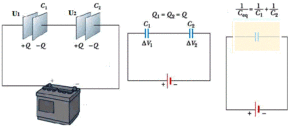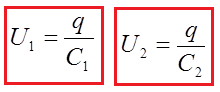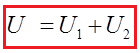When we share the equation with Q, we obtain a mathematical expression for the serial connection of a capacitor: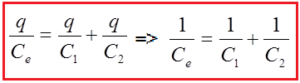Thus, the total capacitance is less than any one of the individual capacitors’ capacitances.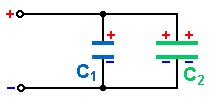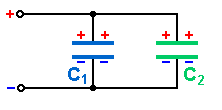When capacitors are connected in parallel, the total capacitance is the sum of the individual capacitors’ capacitances. If two or more capacitors are connected in parallel.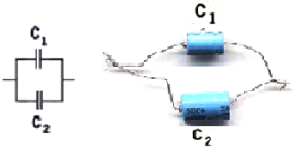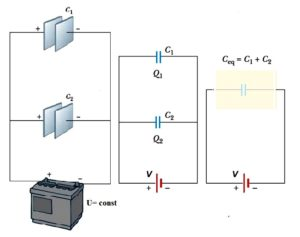The total amount of electricity of this battery is: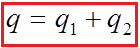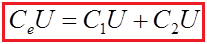When we divide the equation with U, we obtain a mathematical expression for the parallel connection of a capacitor: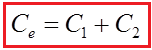Thus, the total capacitance is more than any one of the individual capacitors’ capacitances.

SHARE
Previous articleA PARTY OF FAMOUS PHYSICISTS
Next articleElectric current